#Solutions or Roots of Quadratic Equations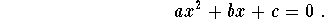A real number x will be called a solution or a root if it satisfies the equation, meaning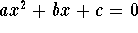. It is easy to see that the roots are exactly the x-intercepts of the quadratic function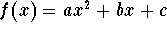, that is the intersection between the graph of the quadratic function with the x-axis.

 a<0 a>0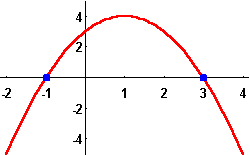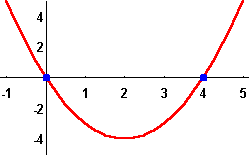Example 1: Find the roots of the equation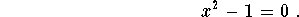Solution. This equation is equivalent to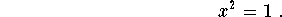Since 1 has two square-roots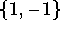, the solutions for this equation are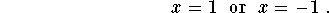Example 2: Find the roots of the equation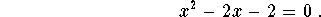Solution. This example is somehow trickier than the previous one but we will see how to work it out in the general case. First note that we haveTherefore the equation is equivalent to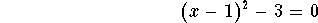which is the same as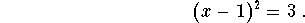Since 3 has two square-roots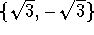, we get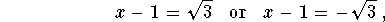which give the solutions to the equation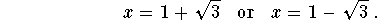We may then wonder whether any quadratic equation may be reduced to the simplest ones described in the previous examples. The answer is somehow more complicated but it was known for a very longtime (to the Babylonians about 2000 B.C. ). Their idea was based mainly on completing the square which we did in solving the second example.[Algebra] [Complex Variables]
[Geometry] [Trigonometry ]
[Calculus] [Differential Equations] [Matrix Algebra]S.O.S MATHematics home page

Do you need more help? Please post your question on our S.O.S. Mathematics CyberBoard.Author: Mohamed Amine Khamsi